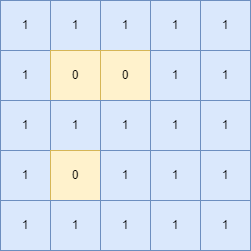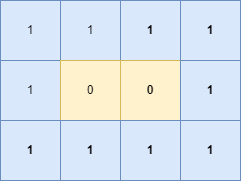Problem title
Difficulty
Avg time to solve

Maximum Difference
Easy
15 mins
Range Sum of BST
Easy
20 mins
Equalize Weights
Moderate
15 mins
Synonymous Sentences
Moderate
20 mins
Stepping Numbers
Moderate
30 mins
Kronecker Product Of Two Matrices
Easy
20 mins
Maximum Number Of Eaten Apples
Moderate
15 mins
Concatenate The Largest Digit
Easy
15 mins
Glowing Bulbs
Hard
30 mins
Mobile Pattern Lock.
Moderate
30 mins1

# Count Islands

Difficulty: MEDIUM

Problem Statement

#### You are given a grid of binary numbers, ‘1’ and ‘0’ where ‘0’ means land and ‘1’ means water. A closed island is a group of ‘0’s surrounded on the 4 sides by water, i.e., ‘1’. Your task is to find the number of islands in the given grid.

##### For Example:
``````You are given ‘grid’ = [[1,1,1 ,1 1], [1, 0, 0, 1 1],[ 1, 1, 1, 1,1], [1, 0, 1, 1, 1],[1, 1, 1, 1, 1]], Here the given grid is
````````````We can clearly see that there are 2 islands. Hence the answer is 2.
``````
##### Input Format:
``````The first line of input contains a single integer ‘T’ representing the number of test cases.

The first line of each test case contains two space-separated integers ‘N’ and ‘M’ representing the row and column of the grid.

The next ‘N’ lines of each test case contain ‘M’ space-separated integers representing the elements of the grid.
``````
##### Output Format:
``````For each test case print a single integer, representing the number of islands in the grid.

Print a separate line of each test case.
``````
##### Constraints:
``````1 <= T <= 10
1 <= N, M <= 10^3
grid[i][i] = 0 or 1

Time Limit: 1 sec
``````
##### Note :
``````You do not need to print anything. It has already been taken care of. Just implement the given function.
``````
##### Sample Input 1:
``````2
5 5
1 1 1 1 1
1 0 0 1 1
1 1 1 1 1
1 0 1 1 1
1 1 1 1 1
3 4
1 1 1 1
1 0 0 1
1 1 1 1
``````
##### Sample Output 1:
``````2
1
``````
##### Explanation:
``````For the first test case, ‘grid’ = [[1,1,1 ,1 1], [1, 0, 0, 1 1],[ 1, 1, 1, 1,1], [1, 0, 1, 1, 1],[1, 1, 1, 1, 1]], Here the given grid is
````````````We can clearly see that there are 2 islands, Hence the answer is 2.

For the second test case, ‘ ‘grid’ = [[1,1,1 ,1], [1, 0, 0, 1 ],[ 1, 1, 1, 1]], Here the given grid is
````````````We can clearly see that there are 1 island. Hence the answer is 1.
``````
##### Sample Input 2:
``````2
2 3
1 0 1
1 0 1
3 3
1 1 1
0 0 0
1 1 1
``````
##### Sample Output 2:
``````0
0
``````Console